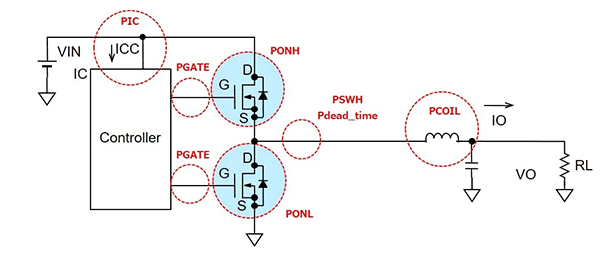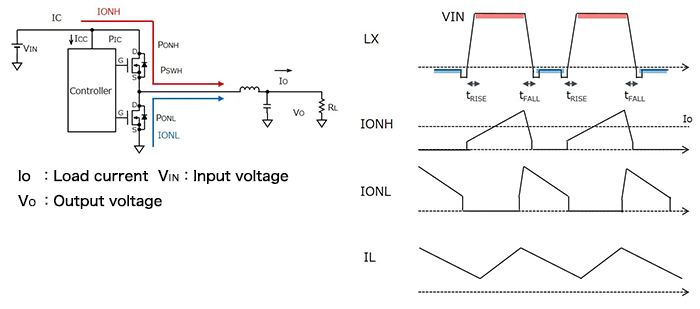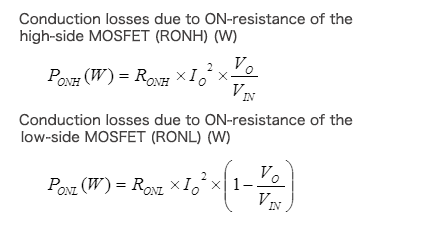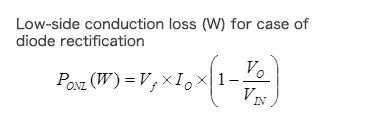Technical Information Site of Power Supply Design

2018.08.09 DC/DC

# Conduction Losses in Synchronous Rectifying Step-Down Converters

Examination of Losses

In the previous section, we identified the different places in a synchronous rectifying step-down converter at which losses occur, and explained that the loss in the converter as a whole is the sum of the losses in different places. From this section, we will study methods to calculate the loss at each point. This time, we consider conduction losses in an output-stage MOSFET, which is a power switch.• PONH: Conduction losses due to the ON-resistance of the high-side MOSFET while on
• PONL: Conduction losses due to the ON-resistance of the low-side MOSFET while on
• PSWH: Switching losses
• PIC: IC self current consumption loss
• PGATE: Gate charge losses
• PCOIL: Conduction losses due to the inductor DCR

Conduction Losses in Output-Stage MOSFETs

The conduction losses in output-stage MOSFETs are due to the ON-resistances of the low-side and high-side MOSFETs (RDS(ON)) while the devices are turned on. Here, the following symbols are used.

PONH: Conduction losses due to the ON-resistance of the high-side MOSFET while on
PONL: Conduction losses due to the ON-resistance of the low-side MOSFET while on

One important parameter representing MOSFET properties is the ON-resistance. A MOSFET always has an ON-resistance. Hence current always flows in a conductor with a resistance, and so it should be obvious that a simple loss occurs as a result.

We derive the conduction loss of a MOSFET. In the circuit diagram below, IONH (red) indicates the current when the high-side MOSFET is turned on, and IONL (blue) is the current when the low-side MOSFET is turned on. The waveform diagram shows a standard example in which LX is the voltage waveform at the switching node, IONH and IONL are the current waveforms that accompany switching, and IL is the inductor current.In synchronous rectification, when the high-side switch is turned on the low-side switch is turned off, and when the low side is on the high side is off. In the switching node waveform, the red parts indicate that IONH is flowing, and the blue parts indicate that IONL is flowing. That is, the power losses due to the currents flowing in the MOSFETs during these periods and the MOSFET ON-resistances result in the respective conduction losses. The equations used for calculation are as follows.As can be seen, the results are equal to the I2R values based on Ohm's laws, multiplied by the fraction of the on-time. The model uses the average current IO.

Incidentally, in the case of diode rectification (asynchronous rectification), the low-side MOSFET in synchronous rectification is merely replaced with a diode, and so losses can be determined using a similar approach. A diode does not have an ON-resistance, and so calculations employ the forward voltage Vf. This voltage Vf is known, and so VI can be used in calculations. In cases where switches are bipolar transistors also, calculations can be performed using VCE, in the same manner as for diodes.What is important in actual calculations is that calculations use, as the value of the ON-resistance, the ON-resistance at the current value IO. A MOSFET data sheet will have a graph of the ON-resistance RDS(ON) versus IDS, and this should be used. The same is true of the Vf of a diode and the VCE of a bipolar transistor.

Next time, we will discuss switching losses.

#### Key Points:

・Conduction losses of a MOSFET of a synchronous rectifying step-down converter are calculated using the ON-resistance, the current while turned on, and the on-time.

This website uses cookies.

By continuing to browse this website without changing your web-browser cookie settings, you are agreeing to our use of cookies.